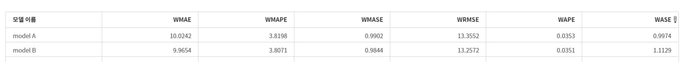## What is the difference of diagnose.setOption('holdout') and forecast.setOption('holdout')??

HI, i'm using Visual Forecasting.

While using atsm package in TSMODEL procedure, I wonder the difference

diagnose.setOption('holdout', 6);

and

forecast.setOption('holdout',6).

**i'm using Auto-forecasting node.

``````proc tsmodel data = &vf_libIn.."&vf_inData"n
%if "&vf_inEventObj" ne "" %then %do;
inobj = (&vf_inEventObj)
%end;
outobj = (
outfor  = &vf_libOut.."&vf_outFor"n
outstat = &vf_libOut.."&vf_outStat"n
outmodelinfo = &vf_libOut.."&vf_outModelInfo"n
)
outlog  = &vf_libOut.."&vf_outLog"n
;
*define time series ID variable and the time interval;
id &vf_timeID interval = &vf_timeIDInterval;

*define time series and the corresponding accumulation methods;
%vf_varsTSMODEL;

*define the by variables if exist;
%if "&vf_byVars" ne "" %then %do;
by &vf_byVars;
%end;

*using the ATSM (Automatic Time Series Model) package;
require atsm;

*starting user script;
submit;

*declaring the ATSM objects;
/*
TSDF:     Time series data frame used to group series variables for DIAGNOSE and FORENG objects
DIAGNOSE: Automatic time series model generation
FORENG:   Automatic time series model selection and forecasting
DIAGSPEC: Diagnostic control options for DIAGNOSE object
OUTFOR:   Collector for FORENG forecasts
OUTSTAT:  Collector for FORENG forecast performance statistics
*/
declare object diagnose(diagnose);
declare object diagspec(diagspec);
declare object dataframe(tsdf);
declare object forecast(foreng);
declare object outfor(outfor);
declare object outstat(outstat);
declare object outmodelinfo(outmodelinfo);

*initialize the tsdf object and assign the time series roles;
rc = dataframe.initialize();
*add independent variables to the tsdf object if there is any;
%if "&vf_indepVars" ne "" %then %do;
%end;
%if "&vf_inEventObj" ne "" or "&vf_events" ne "" %then %do;
declare object ev1(event);
rc = ev1.Initialize();
%end;

*open the diagspec object and enable ESM and ARIMAX model class for diagnose;
rc = diagspec.open();
rc = diagspec.setESM();
rc = diagspec.setARIMAX();
rc = diagspec.setOption('criterion',"&_modelSelection_criteria");
rc = diagspec.close();

*set the diagnose object using the diagspec object and run the diagnose process;
rc = diagnose.initialize(dataframe);
rc = diagnose.setSpec(diagspec);
/*holdout1
rc = diagnose.setOption('holdout', 6);*/
rc = diagnose.run();

*initialize the foreng object with the diagnose result and run model selecting and generate forecasts;
rc = forecast.initialize(diagnose);
rc = forecast.setOption('criterion',"&_modelSelection_criteria");
rc = forecast.setOption('horizon',&vf_horizonStart);
/*holdout2
rc = forecast.setOption('holdout',6);*/
rc = forecast.setOption('minobs.trend',2);
rc = forecast.setOption('minobs.mean',2);
%if "&vf_allowNegativeForecasts" eq "FALSE" %then %do;
rc = forecast.setOption('fcst.bd.lower',0);
%end;
rc = forecast.run();

*collect the forecast and statistic-of-fit from the forgen object run results;
rc = outfor.collect(forecast);
rc = outstat.collect(forecast);
rc = outmodelinfo.collect(forecast);
endsubmit;
quit;

*generate outinformation CAS table;
data &vf_libOut.."&vf_outInformation"n;
set work.sforecast_outInformation;
run;``````

How to works these code on model?

What is the difference with the result of model A and model B?

model A : (add this code) `/*holdout1*/ rc = diagnose.setOption('holdout',6);`

model B : (add this code)`/*holdout2*/ rc = forecast.setOption('holdout',6);<result>`

Thank u 🙂<result>

## Re: What is the difference of diagnose.setOption('holdout') and forecast.setOption('holdout')??

All options for diagnose object determine how to choose best models from model families; all options for forecast objects determine how to choose the best model from model selection list.

For example, in this example code, ARIMA and ESM model families are enabled, so the diagnose.setOption('holdout')  helps choose one model from ARIMA family and one model from ESM family. If you don't set this option explicitly, it will use the default value 0 for holdout. After you already have a list of models, the forecast object forecast.setOption('holdout') helps select the best model among this list. If you don't set this option explicitly, it will use the default value 0 for holdout when making this decision.

So in your case, when you want to use non-zero holdout, you might want to specify this options in both objects to be consistent.

Discussion stats